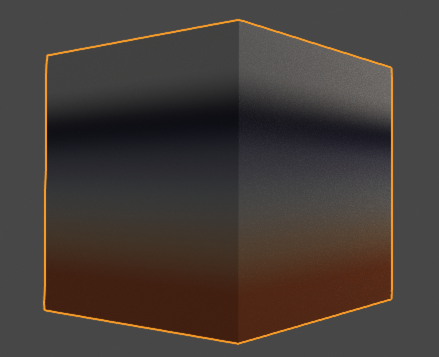# Reviving That Old Pixar Marble

Anybody remember Stephen May’s old RManNotes? I’ve been going through them, and it’s remarkable how similar the old RenderMan Shading Language is to modern Open Shading Language.

Anyway, I have adapted his “rmarble.sl” example to work with OSL in Blender 2.8. Enclosed is my adaptation. I think it looks like quite a nice marble texture.

``````#define MINFILTERWIDTH  1e-7
#define filterwidth_point(p) (max(sqrt(area(p)), MINFILTERWIDTH))

surface rmarble
(
float Veining = 0.5,
float Ks = 0.4,
float Kd = 0.6,
float Ka = 0.1,
float Roughness = 0.1,
color SpecularColour = 1,
output closure color Out = 0
)
{
color surface_colour;
color layer_opac;
point PP;
float width, cutoff, fade, f, turb, maxfreq = 16;
color pattern_colours =
{
color(0.8, 0.2, 0.05),
color(0.8, 0.2, 0.05),
color(0.8, 0.5, 0.3),
color(0.6, 0.594, 0.58),
color(0.3, 0.3, 0.4),
color(0.05, 0.05, 0.1),
color(0.8, 0.8, 0.79),
color(0.8, 0.8, 0.79)
};

surface_colour = 0;

PP = transform("shader", P) * Veining;
width = filterwidth_point(PP);
cutoff = clamp(0.5 / width, 0, maxfreq);

turb = 0;
for (f = 1; f < 0.5 * cutoff; f *= 2)
{
turb += abs(noise("snoise", PP * f)) / f;
} /*for*/
fade = clamp(2 * (cutoff - f) / cutoff, 0, 1);
turb += fade * abs(noise("snoise", PP * f)) / f;
turb *= 0.5; /* to match original rmarble turbulence value */
surface_colour = spline("catmull-rom", turb, pattern_colours);
Out =
surface_colour
*
(
emission() * Ka
+
oren_nayar(N, Roughness) * Kd
+
microfacet_ggx(N, Roughness) * (SpecularColour * Ks)
);
} /*rmarble*/

``````
1 Like

Just a preview for lazy guys.Happy Blending

2 Likes

Thinking about it, all that shader-node stuff is completely unnecessary. Cycles already provides all the shader-node functionality you need. So here is the same thing again, just as a texture node:

``````#define MINFILTERWIDTH  1e-7
#define filterwidth_point(p) (max(sqrt(area(p)), MINFILTERWIDTH))

(
float Veining = 0.5,
output color SurfaceColour = 0
)
{
point PP;
float width, cutoff, fade, f, turb;
float maxfreq = 16;
color pattern_colours =
{
color(0.8, 0.2, 0.05),
color(0.8, 0.2, 0.05),
color(0.8, 0.5, 0.3),
color(0.6, 0.594, 0.58),
color(0.3, 0.3, 0.4),
color(0.05, 0.05, 0.1),
color(0.8, 0.8, 0.79),
color(0.8, 0.8, 0.79)
};

PP = transform("shader", P) * Veining;
width = filterwidth_point(PP);
cutoff = clamp(0.5 / width, 0, maxfreq);

turb = 0;
for (f = 1; f < 0.5 * cutoff; f *= 2)
{
turb += abs(noise("snoise", PP * f)) / f;
} /*for*/
fade = clamp(2 * (cutoff - f) / cutoff, 0, 1);
turb += fade * abs(noise("snoise", PP * f)) / f;
turb *= 0.5; /* to match original rmarble turbulence value */
SurfaceColour = spline("catmull-rom", turb, pattern_colours);
} /*rmarble*/
``````

You can feed the colour output to your choice of shader.

@skuax Looks like Mars, doesn’t it?@ldo hope there ll be a glsl interpreter in script node for eevee one day.

Just for fun, I thought I would pull out those colours from the ramp and convert them to HSV format:

``````shader ramp8
(
float In = 0.0,
output color Out = color(0),
float In0 = 0.000, /* must be < In1 */
float In1 = 0.143, /* must be < In2 */
float In2 = 0.286, /* must be < In3 */
float In3 = 0.429, /* must be < In4 */
float In4 = 0.571, /* must be < In5 */
float In5 = 0.714, /* must be < In6 */
float In6 = 0.857, /* must be < In7 */
float In7 = 1.000
)
{
float xpts = {In0, In1, In2, In3, In4, In5, In6, In7};
color ypts =
{
color("hsv", 0.0333333, 0.9375000, 0.8000000),
color("hsv", 0.0333333, 0.9375000, 0.8000000),
color("hsv", 0.0666667, 0.6250000, 0.8000000),
color("hsv", 0.1166667, 0.0333333, 0.6000000),
color("hsv", 0.6666667, 0.2500000, 0.4000000),
color("hsv", 0.6666667, 0.5000000, 0.1000000),
color("hsv", 0.1666667, 0.0125000, 0.8000000),
color("hsv", 0.1666667, 0.0125000, 0.8000000)
};
float x = splineinverse("catmull-rom", In, xpts);
Out = spline("catmull-rom", x, ypts);
} /*ramp8*/
``````

The ramp looks like this:Further fun fact: this line

``````    turb *= 0.5; /* to match original rmarble turbulence value */
``````

means the range of the “turb” value is only something like [0, 0.5] instead of [0, 1]. This is why only the orange and light hues show up in the pattern, the dark purplish ones do not.

What do you think? Does it look better without that line? Or with some other value for the multiplier (like 0.75)?

I will try it when the road to holidays ll over.Well, after thinking about it, there was some educational value in converting the code from RSL to OSL, but apart from that, I would say the result is not worth using. You can get as good, or better, results, using Cycles’ “Wave” texture node, applying a bit of Distortion and playing with the Scale and Detail Scale settings to get a nice pattern, then using the result to drive a colour ramp or RGB Mix setup to get a more interesting colour scheme.

Another one for the museum, folks …